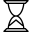## Description

Currently we generate derived data for annotations, to save clients from having to do it themselves.

The ICU ldml converter also generates derived data for ICU, where the derived data format would be more suitable for processing.

We might want to consider doing a bit more derived data. That could have two benefits:

1. making the ICU conversion easier,r

2. providing a more "processable" format for clients other than ICU (who might want to use that format).
Sometimes the format would be very specific to ICU, but I think often it would be more generally applicable.3.

3. Allow us to do more extensive consistency and completeness testing in the CLDR framework between the original and derived data.

For example, the unit preferences can be preprocessed to have a mapping from regions to ids-for-regions-that-behave-the-same, allowing for faster, more compact processing. For comparison, here is the inverse of that for v37 (id to regions).

0=[AG, AI, AO, AU, BA, BG, BH, BM, BN, BW, BY, CH, CM, CZ, DM, EE, FJ, GD, HR, HU, IE, IM, IS, KE, KN, KW, KZ, LC, LI, LT, LU, LV, ME, MG, MK, MO, MS, MT, MU, MZ, NA, NZ, OM, PG, RS, SG, SI, SK, TC, TO, UA, UG, VC, VG, VU, ZA]
1=[AT, BE, FR, ID, PT]
2=[BR]
3=[BS, BZ, KY, PR, PW]
4=[CA]
5=[CN, DK, VN]
6=[DE]
7=[DZ, ES, JO, SA]
8=[EG]
9=[FI]
10=[GB]
11=[HK]
12=[IL]
13=[IN]
14=[IT, TR]
15=[JP]
16=[KR]
17=[MX]
18=[MY]
19=[NL]
20=[NO]
21=[PL]
22=[RU]
23=[SE]
24=[TH]
25=[US]
26=[other]

None

None

Unassigned

Mark Davis

None

None

None

None

None

None

None

None

None

## PriorityTBD
Configure